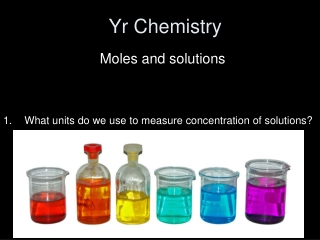DownloadDownload PresentationYr Chemistry

# Yr Chemistry

Download Presentation## Yr Chemistry

- - - - - - - - - - - - - - - - - - - - - - - - - - - E N D - - - - - - - - - - - - - - - - - - - - - - - - - - -
##### Presentation Transcript

1. Yr Chemistry What units do we use to measure concentration of solutions? Moles and solutions

2. Moles and solutions Objective: to know how we can calculate concentration Outcomes: • carry out calculations, using amount of substance in mol, involvingsolution volume and concentration • deduce stoichiometric relationships from calculations • use the terms concentrated and dilute as qualitative descriptions for the concentration of a solution.

3. How can we measure moles of a dissolved substance? • In chemistry we are very often reacting dissolved substances. • In a solution there is the solute and the solvent. • Usually we are interested in how much solute we have. • The amount of solute that there is in a certain volume of solvent is called the …? Concentration!

4. The concentration of a solution is how many moles are dissolved per dm3 (1 litre) of solution. The units are mol dm-3

5. Concentrations • Concentrations are measured in: moles per decimetre cubed - mol.dm-3 • A chemist dissolves 0.25 moles of NaCl (14.625g) in one litre of water. • What is the concentration? • 0.25 mol.dm-3 • How much NaCl would have to be dissolved in 250ml of water to get the same concentration?

6. Molarities • Concentrations are traditionally expressed in molarities. • A solution that is 1 mol.dm-3is described as being 1 molar (1M) • A solution that is 0.25 mol.dm-3is described as being: 0.25M • This is the terminology that you usually see on the side of bottles of chemicals.

7. Calculating concentration • Find out how many moles of solute you have. • Find out how many dm-3 of solution you have. • Perform the simple calculation:

8. Calculating concentration • Find out how many moles of solute you have. Moles = Mass Mr • Find out how many dm3 of solution you have. Volume in dm3 = volume in cm31000

9. Calculating concentration • Perform the simple calculation:concentration(mol.dm-3) = moles . volume (dm3)

10. Practise • Starters for 10 • Page 55 edexcel revision guide • OCR text book page 17

11. Mass concentration The concentration of a solution can also be measured by how many grams of a substance are dissolved per dm3 of the solution. Mass concentration is expressed as the mass of solute in 1dm-3 of solution: concentration(g.dm-3) = mass(g) . volume (dm3)

12. Practise • Complete question 3 on page 17 ocr book